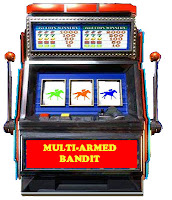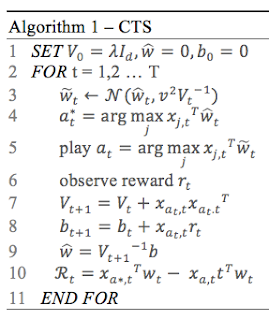## Sunday, October 20, 2019

### Contextual Thompson sampling: Theory

This post introduces the concept of multi-armed bandit (a.k.a. K-armed bandit) and the Thompson sampling for contextual bandit. The next post deals with the actual implementation of the contextual Thompson sampling in Apache Spark.

## Multi-arm Bandit problem

The multi-armed bandit problem is a well-known reinforcement learning technique,  widely used for its simplicity. Let's consider a  slot machine with n arms (bandits) with each arm having its own biased probability distribution of success. In its simplest form pulling any one of the arms gives you a  reward of either 1 for success, or 0 for failure. Our objective is to pull the arms one-by-one in sequence such that we maximize our total reward collected in the long run.Dual-arm bandit

Data scientists have developed several solutions to tackle the multi-armed bandit problem for which the 3 most common algorithms are
• Epsilon-Greedy
• Upper confidence bounds
• Thompson sampling
Epsilon-greedy
The Epsilon-greedy is the simplest algorithm to address the  exploration-exploitation trade-off.  During exploitation, the lever with highest known payout is always pulled. However, from time to time (fraction ε with  ε < 0.1) the algorithm selects a random arm to 'explore' other arms which payout is relatively unknown. The arms with known or proven highest payout are pulled (1-ε of the time)

Upper Confidence Bound (UCB)
This solution is sometimes referred as Optimism in the Face of Uncertainty principle: It assumes that the unknown mean payoffs of each arm will be as high as possible, based on historical data.

Thompson Sampling
The Thompson sampling algorithm is fundamentally a Bayesian optimization technique which core principle known as probability matching strategy can be summed as ‘play an arm according to its probability of being the best arm’.(i.e. the number of pulls for a given lever should match its actual probability of being the optimal lever)

The following diagram illustrates the difference between the upper confidence bounds and the Thompson sampling.Confidence Bounds for Multi-Armed bandit problem

## Context anyone?

The techniques described above do not make any assumption on the environment or the agent who selects the arms. There are two scenario:
• Context-free bandit: The selection of the arm with the highest rewards depends solely on the past history (prior) reward (success and failure). Such history can be modeled using a Bernoulli distribution. For instance, the probability to get a '6' playing dice does not depend on the player.
• Contextual bandit: The state of the environment (a.k.a. context) is used as an input (or model) to the selection of the arm with the highest predicted reward. The algorithm observes a new context (state), choose one action (arm), and observes an outcome (reward). In ad targeting, the selection of a banner or insert to be displayed on a web site depends on the prior rewards history associated to the demographic data of the user.
Any of the techniques applied to the multi-armed bandit can be used with and without context. The following sections focus on the contextual multi-arm bandit problem.

## Contextual Thompson sampling (CTS)

Let's dive into the key characteristics of the Thompson sampling
• We assume the prior distribution on the parameters of the distribution (unknown) of the reward for each arm.
• At each step, t, the arm is selected according to the posterior probability to be the optimal arm.
The components of the contextual Thompson sampling are
1. Model of parameters w
2. A prior distribution p(w) of the model
3. History H consisting of a context x and reward r
4. Likelihood or probability p(r|x, w) of a reward given a context x and parameters w
5. Posterior distribution computed using naïve Bayes$p(\widetilde{w}|H)=p(H|\widetilde{w}).p(\widetilde{w})/p(H))$

But how can we model a context?

Actually, a process to select the most rewarding arm is actually a predictor or a learner. Each predictor takes the context, defines as a vector of features and predicts which arm will generate the highest reward.

The predictor is a model that can be defined as
- Linear model
- Generalized linear model (i.e. logistic)
- Neural network

The algorithm is implemented as a linear model (weights w) for estimating the reward from a context x  as $w^{T}.x$
The ultimate objective for any reinforcement learning model is to extract a policy which quantifies the behavior of the agent. Given a set X of context xi and a set A of arms, the policy is defined by the selection of an arm given a context x
$\pi : X\rightarrow A$Contextual Thompson Sampling Algorithm

The sampling of the normal distribution (line 3) is described in details in the post Multivariate Normal Sampling with Scala. The algorithm computes the maximum reward estimation through sampling the normal distribution (line 4) and play the arm a* associated to it (line 5).
The parameters V and b are updated with the estimated value (line 7 and 8) and the actual reward is computed (line10) after the weights of the context are updated (line 9)

The next post describes the implementation of the contextual Thompson sampling using Scala, Nd4j and Apache Spark.

## Monday, July 22, 2019

### Multivariate Normal sampling with Scala and ND4j

Target audience: Intermediate

This post describes the implementation of the multivariate normal sampling using ND4j.

The multi-variate normal sampling function is used in various machine learning techniques such as Markov chains Monte Carlo (MCMC) or contextual multi-armed bandit.
The implementation of the multivariate normal relies on data vectorization, technique well-known to Python developers and data scientists alike.

Note: The post requires some knowledge of data vectorization (numpy, datavec, ND4j..) as well as Scala programming language.

Vectorization
Python Numpy is a well-known and reliable vectorized linear algebra library which is a foundation of scientific (SciPy) and machine learning (Sciktlearn) libraries. No serious data scientific projects can reasonably succeeds with the power and efficiency of numpy.
The vectorization of datasets is the main reason behind the performance of machine learning models (training and prediction) build in Python.

Is there a similar linear algebra library, supporting vectorization, available to Scala and Spark developers? Yes, ND4j

ND4j, BLAS and LAPACK
ND4j library replicates the functionality of numpy for Java developers. ND4j can be downloaded as an independent library or as component of the deep learning library, deeplearning4j. It leverages the BLAS and LAPACK libraries.
From a Java developer perspective, the data represented by an NDArray is stored outside of the Java Virtual Machine. This design has the following benefits:
• Avoid taxing the Garbage Collector
• Interoperability with high-performance BLAS libraries such as OpenBlas
• Allow number of array elements exceeds Int.MaxValue
The BLAS (Basic Linear Algebra Subprograms) are functions performing basic vector and matrix operations. The library is divided in 3 levels:
• Level 1 BLAS perform scalar, vector and vector-vector operations,
• Level 2 BLAS perform matrix-vector operations
• Level 3 BLAS perform matrix-matrix operations.
OpenBLAS is an optimized Basic Linear Algebra Subprograms (BLAS) library based on GotoBLAS2,  a C-library of linear algebra supporting a large variety of micro-processor. Its usage is governed by the BSD license.
LAPACK are Fortran routines for solving systems of simultaneous linear equations, least-squares solutions of linear systems of equations, eigenvalue problems, and singular value problems and matrix factorizations.

Implicit ND4j array conversion
The first step is to create a implicit conversation between ND4j and Scala data types.
The following code convert an array of double into a INDArray using org.nd4j.linalg.factory.Nd4j java class and its constructor create(double[] values, int[] shape)
• In case of a vector, the shape is defined in scala as  Array[Int](size_vector)
• In case of a matrix, the shape is defined as Array[Int](numRows, numCols).
The following snippet implement a very simple conversion from a Scala array to a INDArray

 1 2 3 4 5 6 @throws(clazz = classOf[IllegalArgumentException]) implicit def double2NDArray(values: Array[Double]): INDArray = { require(values.nonEmpty, "ERROR: ND4, conversion ...") Nd4j.create(values, Array[Int](1, values.length)) } 

Multivariate Normal distribution implementation
The sampling of a multivariate normal distribution is defined by the formula
$\mathbb{N}(\mu, \Sigma )=\frac{1}{\sqrt{(2\pi)^n \left | \Sigma \right |}} e^{\frac{1}{2}(x-\mu)^T {\Sigma '}^{-1}(x-\mu)}$ A generalized definition adds a very small random perturbation factor r |r| <= 1 on the variance value (diagonal of the covariance matrix) $\Sigma ' = \Sigma + r.I$ Sigma is the covariance matrix and the mu is the mean value.
The computation of the multivariate normal sampling can be approximated by the Cholesky decomposition. In a nutshell, the Cholesky algorithm decompose a positive-definite matrix into a product of two matrix
• lower triangle matrix
• transposition of its conjugate
It serves the same purpose as the ubiquitous LU decomposition with less computation. $\mathbb{N}(\mu, \Sigma) \sim \mu + Chol(\Sigma).Z^T$ where $L=Chol(\Sigma)$ and $L.L^T=\Sigma$. The vector Z is a multivariate normal noise $Z= \{ z_i | z_i=N(0, 1)\}$
The following implementation relies on the direct invocation of LAPACK library function potrf. The LAPACK functionality are accessed through the BLAS wrapper getBlasWrapper.

 1 2 3 4 5  final def choleskyDecomposition(matrix: INDArray): INDArray = { val matrixDup = matrix.dup Nd4j.getBlasWrapper.lapack.potrf(matrixDup, false) matrixDup } 

Note that the matrix is duplicated prior to the LAPACK function call as we do not want to alter the input matrix.
Let's implement the multivariate Normal sampler with perturbation using the Cholesky decomposition.

References